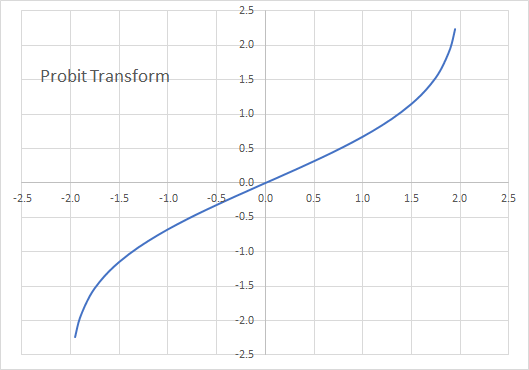# PROBIT - Probit Transform

Computes the Probit transformation and its inverse.

## Syntax

PROBIT(X, Lo, Hi, Return)
X
is the real number for which we compute the transformation. X must be between zero and one (exclusive).
Lo
is the x-domain lower bound. If missing, Lo is assumed to be 0.
Hi
is the x-domain upper bound. If missing, Hi is assumed to be 1.
Return
is a number that determines the type of return value: 0 (or missing) = Probit, 1 = Inverse Probit.
Return Description
0 or omitted Probit Transform
1 The inverse of Probit transform

## Remarks

1. The X value(s) must be between Lo and Hi (exclusive).
2. The Probit function is the quantile function associated with the standard normal distribution.
3. The Probit function is commonly used for parameters that lie in the unit interval (i.e., $x\in({0,1})$).
4. Numerical values of X close to 0 or 1 or out of range result in #VALUE! or #N/A.
5. The Probit function is defined as the inverse cumulative distribution function (CDF):
$$y=\textit{Probit}(x)=\Phi^{-1}(x)$$ And,
$$x=\textit{Probit}^{-1}(y)=\Phi(y)=\frac{1}{\sqrt{2\pi}}\int_{-\infty}^{y}e^{\frac{-Z^2}{2}}dz$$ Where:
• $x_{t}$ is the input value of the input time series at time $t$. X must be between 0 and 1 (exclusive).
• $y_{t}$ is the transformed Logit value at time $t$.
• $\Phi(.)$ is the standard Gaussian cumulative density function.
• $\textit{Probit}^{-1}(y)$ is the inverse Probit transformation.
6. To support a generic interval (a, b), we perform the following mapping:
$$z = \frac{x - a}{b - a}$$ So, the revised transform function is expressed as follows:
$$y=\Phi^{-1}(\frac{x - a}{b - a}) \Rightarrow \Phi(y)=\frac{x - a}{b - a}$$ The inverse transform is expressed as follows:
$$x = a+(b - a)\times\Phi(y)$$
7. The transform first order derivative is calculated as follows:
$$\frac{dy}{dx}=\frac{1}{(b - a)\phi(y)}=\frac{1}{(b - a)\phi(\Phi^{-1}[\frac{x - a}{b - a}]})$$ Where:
$\phi(.)$ is the standard Gaussian probability density function.
8. The transform first order derivative (i.e., $\frac{dy}{dx}$) is positive for all x-values and goes to infinity as x approaches the interval endpoints.
9. In essence, the Probit function converts a bounded x-range from (Lo, Hi) to $(-\infty,\infty)$.10. The Probit function accepts a single value or an array of values for X.

## Examples

Example 1:

1
2
3
4
5
6
7
8
9
10
11
12
13
14
15
16
17
18
19
20
21
22
23
24
25
A B C D
Date Data PROBIT Inv-PROBIT
January 10, 2008 0.66 0.64 0.66
January 11, 2008 0.02 -3.99 0.02
January 12, 2008 0.54 0.18 0.54
January 13, 2008 0.21 -1.34 0.21
January 14, 2008 0.73 1.02 0.73
January 15, 2008 0.37 -0.52 0.37
January 16, 2008 1.00 6.25 1.00
January 17, 2008 0.42 -0.32 0.42
January 18, 2008 0.99 5.27 0.99
January 19, 2008 0.04 -3.22 0.04
January 20, 2008 0.23 -1.20 0.23
January 21, 2008 0.31 -0.79 0.31
January 22, 2008 0.69 0.82 0.69
January 23, 2008 0.37 -0.54 0.37
January 24, 2008 0.78 1.28 0.78
January 25, 2008 0.30 -0.86 0.30
January 26, 2008 0.97 3.45 0.97
January 27, 2008 0.91 2.29 0.91
January 28, 2008 0.92 2.40 0.92
January 29, 2008 0.88 1.97 0.88
January 30, 2008 0.14 -1.78 0.14
January 31, 2008 0.06 -2.81 0.06
February 1, 2008 0.19 -1.42 0.19
February 2, 2008 0.61 0.46 0.61